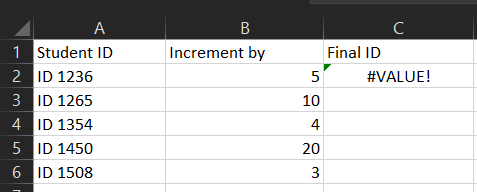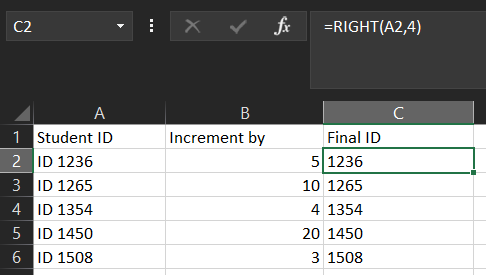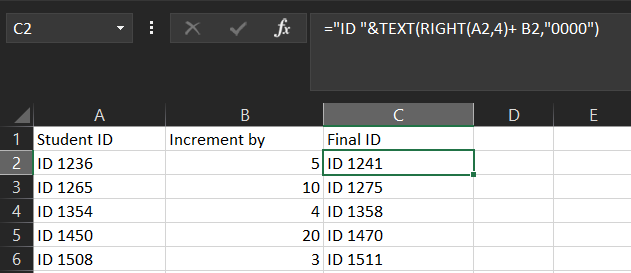Related Articles

# How to Combine Excel Functions in a Formula?

• Difficulty Level : Medium
• Last Updated : 27 May, 2021

We all use Excel for some small computations in data but excel also provides the functionality to combine these small functions and make a formula for manipulating the data.

For example, let’s say that in school we need to increment the ID numbers of students by a number but the ID’s have some text in the beginning and as demonstrated here excel does not allow adding numbers to the text and therefore if we simply use the add function, it will show as the value is invalid as demonstrated here.This is where combining functions and making a formula comes into play. In this example, we will use the split function, then manipulate the data using add function, and then again rejoin the values to get the final result.

We will first use the right function to extract the numbers from column A using the formula =RIGHT(B2,4).

This gives the four digits from the right on which we can now perform the increment function as well.Now that we have the digits we can perform the operations required and then again join it with the text which in this case is ID. As we add the increment value excel automatically converts the result into text but since we also want to concatenate the text (ID) we will have to convert the value into text using the TEXT function.`="ID "&TEXT(RIGHT(A2,4)+ B2,"0000")                `

Just like this many formulas can be made according to the result required also the things that are important are the correct balancing of brackets for all the functions and also checking that the correct numbers of parameters are passed in each function.

My Personal Notes arrow_drop_up